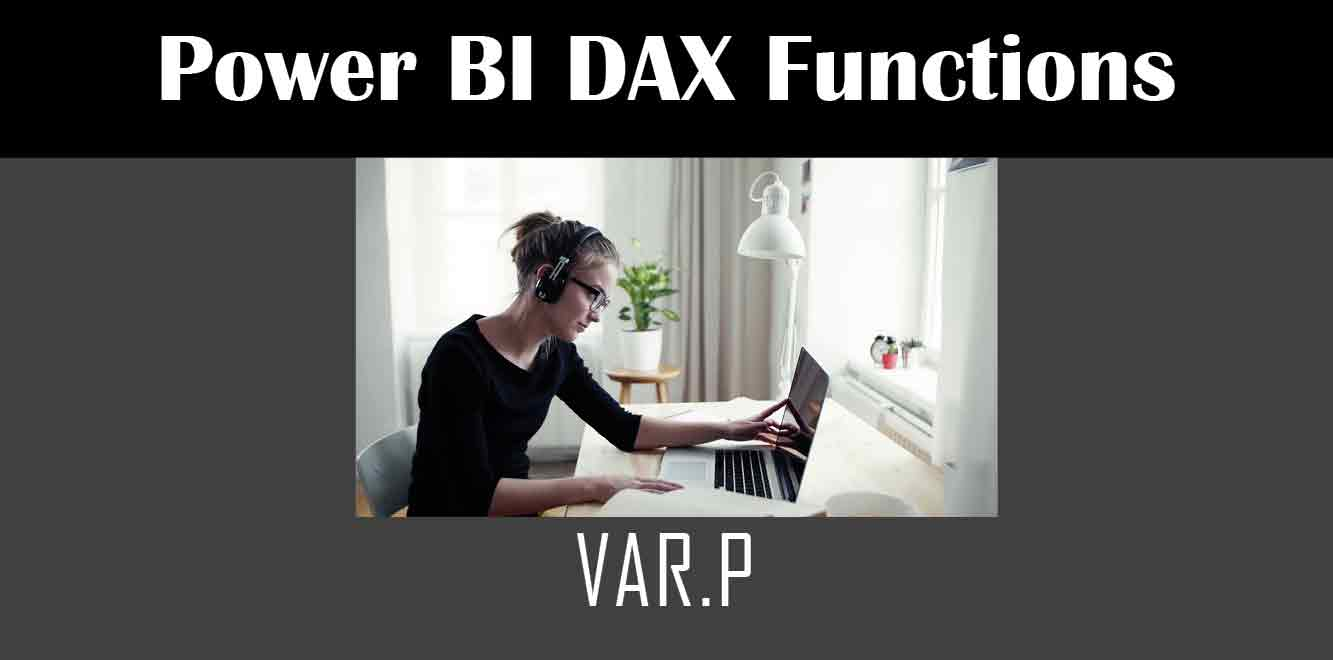# VAR.P

## Y## Syntax of VAR.P function

The syntax of the VAR.P function is as follows:

VAR.P(❰expression❱)

``` The ❰expression❱ represents the column or measure for which we want to calculate the variance of the population. Example Let's take an example to understand how to use the VAR.P function in Power BI. Suppose we have a sales data table that contains the following columns: - Product - Date - Sales We want to calculate the variance of sales for all products. To do this, we can create a measure using the VAR.P function as follows: ```

Sales Variance = VAR.P(Sales)

``` This measure will calculate the variance of sales for all products in the sales data table. Steps to use VAR.P function in Power BI Now let's go through the steps to use the VAR.P function in Power BI: 1. Open Power BI Desktop. 2. Load the data into Power BI that you want to analyze. 3. Click on the "New Measure" button under the "Modeling" tab. 4. Enter a name for the measure. 5. Write the VAR.P function with the expression for which you want to calculate the variance of the population. 6. Click on the "Enter" button to create the measure. Best practices for using VAR.P function Here are some best practices to keep in mind when using the VAR.P function in Power BI: - Always use the VAR.P function with a population to get accurate results. - Use the VAR.P function to calculate the variance for multiple columns or measures. - Use the VAR.P function with the AVERAGE function to calculate the standard deviation of a population. The VAR.P function is a powerful DAX function in Power BI that helps to calculate the variance of a population. In this article, we went through the syntax of the VAR.P function, an example of how to use the VAR.P function in Power BI, steps to use the VAR.P function, and best practices for using the VAR.P function. By following these guidelines, you can leverage the full potential of the VAR.P function in Power BI for your data analysis needs. Power BI DAX Training Courses by G Com Solutions (0800 998 9248)Power BI DAX Intensive Training Course £1,050.00 – £26,550.00 Select optionsContinue Loading DonePower BI DAX Introduction £395.00 – £9,750.00 Select optionsContinue Loading DonePower BI DAX Intermediate £395.00 – £9,750.00 Select optionsContinue Loading DonePower BI DAX Advanced £395.00 – £9,750.00 Select optionsContinue Loading Done Upcoming Courses Contact Us Subject Your Name (required) Company/Organisation Email (required) Telephone Training Course(s) Power BI Intensive TrainingPower BI introduction Power BI IntermediatePower BI AdvancedDAXPower Query MPower BI CertificationPower BI AdministrationPower PlatformPower AutomatePower AppsOTHER Your Message Upload Example Document(s) (Zip multiple files) ```
``` ```
``` ```
``` ```
``` ```
``` ```